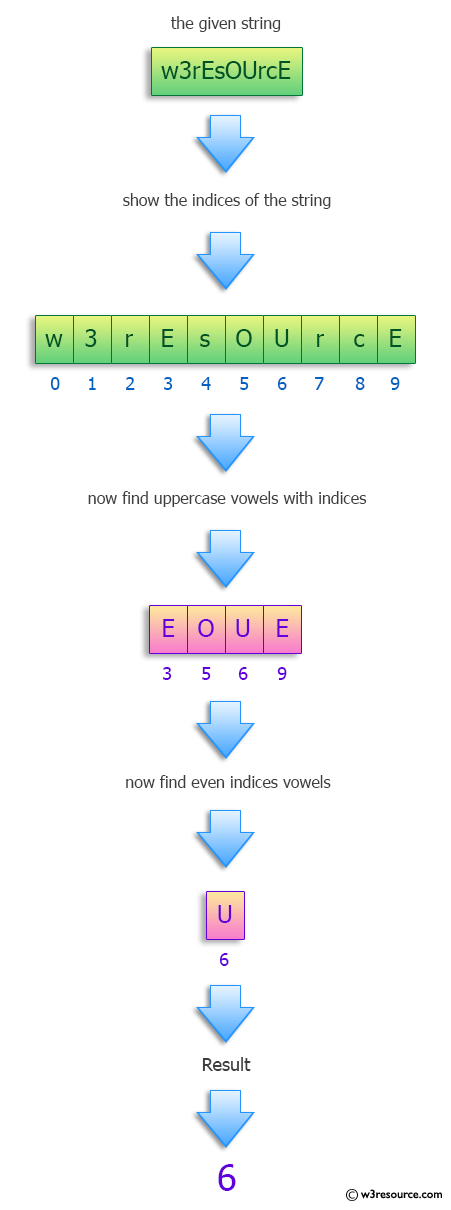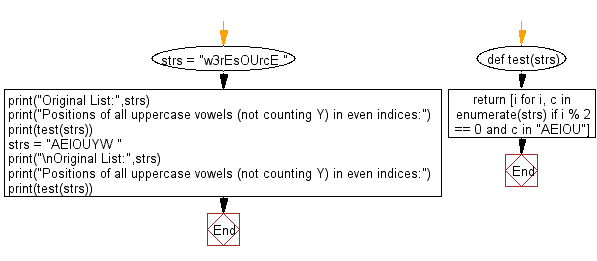﻿ Python: Find the positions of all uppercase vowels (not counting Y) in even indices - w3resource# Python: Find the positions of all uppercase vowels (not counting Y) in even indices

## Python Programming Puzzles: Exercise-33 with Solution

Write a Python program to find the positions of all uppercase vowels (not counting Y) in even indices of a given string.

From Wikipedia:
A vowel is a syllabic speech sound pronounced without any stricture in the vocal tract. Vowels are one of the two principal classes of speech sounds, the other being the consonant. Vowels vary in quality, in loudness and also in quantity. There are six vowels in the English language: a, e, i, o, u and sometimes y.

```Input:
w3rEsOUrcE
Output:


Input:
AEIOUYW

Output:
[0, 2, 4]
```

Pictorial Presentation:Sample Solution:

Python Code:

``````#License: https://bit.ly/3oLErEI

def test(strs):
return [i for i, c in enumerate(strs) if i % 2 == 0 and c in "AEIOU"]
strs = "w3rEsOUrcE "
print("Original List:",strs)
print("Positions of all uppercase vowels (not counting Y) in even indices:")
print(test(strs))
strs = "AEIOUYW "
print("\nOriginal List:",strs)
print("Positions of all uppercase vowels (not counting Y) in even indices:")
print(test(strs))
``````

Sample Output:

```Original List: w3rEsOUrcE
Positions of all uppercase vowels (not counting Y) in even indices:


Original List: AEIOUYW
Positions of all uppercase vowels (not counting Y) in even indices:
[0, 2, 4]
```

Flowchart:## Visualize Python code execution:

The following tool visualize what the computer is doing step-by-step as it executes the said program:

Python Code Editor :

Have another way to solve this solution? Contribute your code (and comments) through Disqus.

What is the difficulty level of this exercise?

Test your Programming skills with w3resource's quiz.

﻿

## Python: Tips of the Day

Clamps num within the inclusive range specified by the boundary values x and y:

Example:

```def tips_clamp_num(num,x,y):
return max(min(num, max(x, y)), min(x, y))
print(tips_clamp_num(2, 4, 6))
print(tips_clamp_num(1, -1, -6))
```

Output:

```4
-1
```